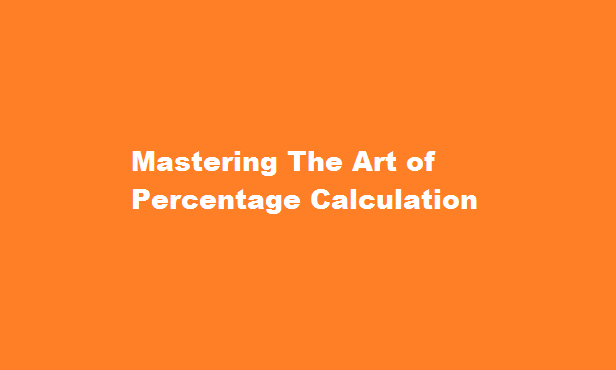December 4, 2023
[stock-market-ticker]

# Mastering The Art of Percentage CalculationContents

## Introduction

Percentage calculations are a fundamental aspect of mathematics that we encounter in various aspects of our daily lives, from analysing data to making financial decisions. Understanding how to calculate percentages accurately is essential for effective problem-solving and decision-making. In this article, we will delve into the concept of percentages, explore different methods for calculating them, and provide practical examples to help you master the art of percentage calculation.

## Understanding Percentages

Before we delve into the calculations, let’s ensure we have a clear understanding of what percentages represent. A percentage is a way of expressing a fraction or a proportion out of 100. It is often denoted by the symbol “%.” Essentially, a percentage helps us compare one value to a whole. For instance, if we say that 75% of students passed an exam, it means 75 out of every 100 students achieved a passing grade.

Now, let’s explore three common types of percentage calculations: finding the percentage of a number, finding the number when given the percentage, and finding the percentage change.

## Finding the Percentage of a Number

To calculate the percentage of a number, follow these steps

• Convert the percentage to a decimal by dividing it by 100. For example, 25% becomes 0.25.
• Multiply the decimal by the number you want to find the percentage of. For example, if you want to find 25% of 80, multiply 0.25 by 80. The result is 20.

## Finding the Number When Given the Percentage

To determine the original number when given a percentage, follow these steps

• Convert the percentage to a decimal.
• Divide the given value by the decimal obtained in Step 1. For example, if you have 20 and want to find the number that represents 25% of it, divide 20 by 0.25. The result is 80.
Also Read  Mastering The Art of Cracking MNC Interviews

## Finding the Percentage Change

Percentage change measures the relative difference between two values. To calculate the percentage change, follow these steps

• Determine the difference between the old value and the new value.
• Divide the difference by the old value and multiply by 100 to express it as a percentage.

For example, if a stock’s value increased from \$50 to \$70, the difference is \$20. Divide \$20 by \$50 and multiply by 100 to find a 40% increase.

### How do you find 25% of 80?

25% of 80 is 20. To calculate the percent of a number: Convert the percent to a decimal by moving the decimal left 2 two places. Multiply the decimal from above and the number to get the result.

Also Read  What Is Sleep And Why Is It Important?

### How do you calculate percentages per question?

You can find your test score as a percentage by dividing your score by the total number of points, then multiplying by 100.

## Conclusion

Calculating percentages is a vital skill that empowers us to make informed decisions in various fields. Whether you are comparing data sets, analysing discounts, or tracking changes over time, understanding how to calculate percentages accurately is essential. By following the methods outlined in this article and practising with real-life examples, you can strengthen your ability to work with percentages. Remember to convert percentages to decimals when necessary and approach each problem step by step. With practice, you will master the art of percentage calculation and apply it confidently in your daily life.